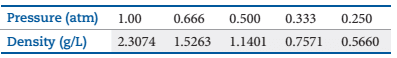### Create an Account

Home / Questions / The density of a gas of unknown molar mass was measured as a function of pressure at 0 °C,...

# The density of a gas of unknown molar mass was measured as a function of pressure at 0 °C, as in the table that follows. (a) Determine a precise molar mass for the gas

The density of a gas of unknown molar mass was measured as a function of pressure at 0 °C, as in the table that follows. (a) Determine a precise molar mass for the gas. [Hint: Graph d/P versus P.] (b) Why is d/P not a constant as a function of pressure?Aug 23 2021 View more View LessSubscribe To Get Solution